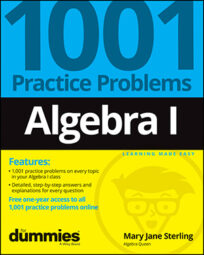##### Algebra I: 1001 Practice Problems For Dummies (+ Free Online Practice)One of the most common goals in algebra I is solve an equation. Solving an equation means to identify the number or numbers you can replace the variable with to make a true statement. You'll find factoring and the multiplication property of zero to be your first approach, and then you'll also have the quadratic formula to use on some of the more challenging second degree equations. Polynomials can be solved using synthetic division to help with the factoring.

## Linear Equations

Linear equations are of the form ax + b = c, where x is some variable, and a, b, and c are real numbers. To solve a linear equation, you perform a series of opposites:

• If a number is added to the term containing x, you subtract that number from both sides of the equation.

• If a number is subtracted from the term containing the variable, you add.

• If a number multiplies the variable, you divide.

• If a number divides the variable, you multiply.

Just be sure that whatever you do to one side of the equation, you also do to the other side. Think of the equation as two expressions pivoting on either side of a balance scale: You need to keep the sides at the same weight.

A quadratic equation has the form ax2 + bx + c = 0. The equation can have two solutions, only one solution (a double root), or no solutions among the real numbers. Where no real solution occurs, imaginary numbers are brought into the picture. Quadratic equations are solved most easily when the trinomial factors, but the quadratic formula is also a nice means to finding solutions.

## Polynomials

A polynomial is a smooth curve that goes on and on forever, from negative infinity to positive infinity. To solve a polynomial means to set the equation equal to 0 and determine which, if any, numbers create a true statement. Any numbers satisfying this equation give you important information: They tell you where the graph of the polynomial crosses or touches the x-axis.